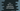# How to use logical OR operator,|| operator in C++## C++ ‘OR’ operator or ’||’ operator explanation with example:

OR logical operator can be used in C++ by using or keyword or ||. In this post, we will learn how to use this operator with examples.

### Definition of logical or:

Logical OR is defined as like below:

``first_operand or second_operand``

or we can also use ||:

``first_operand || second_operand``

It works on two operands.

It will evaluate to True if one of the two operators is true. If both are false, it will evaluate to False.

In C++, it returns 1 for true and 0 for false.

### Example of logical or:

Let’s write one C++ program that uses logical or to find the result:

``````#include <iostream>

using namespace std;

int main()
{
int v = 2;

cout << (v > 10 || v < 5) << endl;
cout << (v < 5 || v > 10) << endl;
cout << (v > 10 || v > 5) << endl;

return 0;
}``````

For this example,

• For the first one, the first operand is false, the second operand is true. So it will be true
• For the first one, the first operand is false, the second operand is true. So it will be true
• For the first one, the first operand is false, the second operand is false. So it will be false

It will print the below output:

``````1
1
0``````

1 represents true and 0 represents false.

We can also use or instead of || in this program:

``````#include <iostream>

using namespace std;

int main()
{
int v = 2;

cout << (v > 10 or v < 5) << endl;
cout << (v < 5 or v > 10) << endl;
cout << (v > 10 or v > 5) << endl;

return 0;
}``````

It will give similar output.

## C++ example program of OR with user given value:

Let’s try one example of OR with user-input values. This program will ask the user to enter a number. It will check if it is divisible by 3 or 5 or not and it will print one message.

``````#include <iostream>

using namespace std;

int main()
{
int num;

cout << "Enter a number: " << endl;
cin >> num;

if (num % 3 == 0 or num % 5 == 0)
{
cout << num << " is divisible by 3 or 5" << endl;
}
else
{
cout << num << " is not divisible by 3 or 5" << endl;
}

return 0;
}``````
• This program is asking the user to enter a number. It reads and store that in the variable num.
• The if block is checking if the number is divisible by 3 or 5.
• If it is divisible by 3 or 5, it will enter to the if block, else it will enter to the else block.
• Based on the result, it will print different message to the user.

If you run this progarm, it will print output as like below:

``````Enter a number:
33
33 is divisible by 3 or 5

Enter a number:
10
10 is divisible by 3 or 5

Enter a number:
13
13 is not divisible by 3 or 5``````